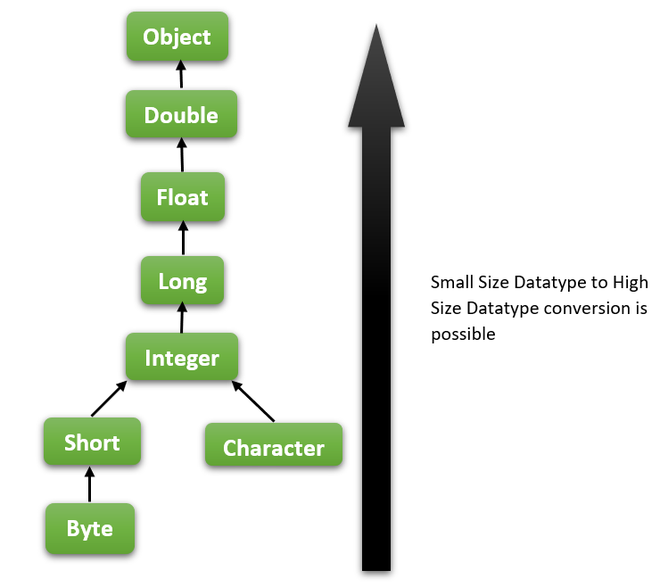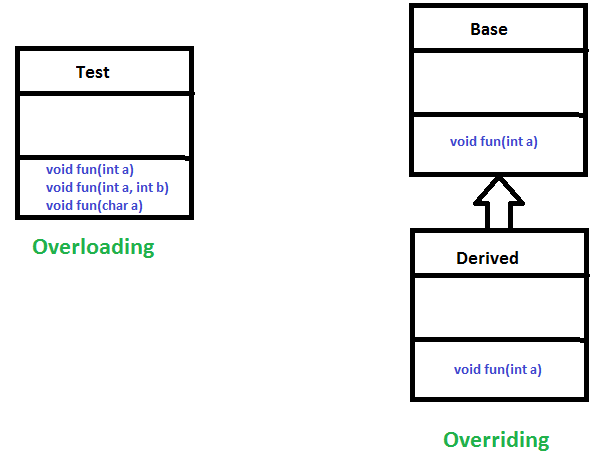Open in App
Not now

• Difficulty Level : Easy
• Last Updated : 07 Sep, 2022

Method Overloading allows different methods to have the same name, but different signatures where the signature can differ by the number of input parameters or type of input parameters, or a mixture of both.

Method overloading is also known as Compile-time Polymorphism, Static Polymorphism, or Early binding in Java. In Method overloading compared to parent argument, child argument will get the highest priority.

## Java

 `// Java program to demonstrate working of method ` `// overloading in Java ` ` `  `public` `class` `Sum { ` ` `  `    ``// Overloaded sum(). This sum takes two int parameters ` `    ``public` `int` `sum(``int` `x, ``int` `y) { ``return` `(x + y); } ` ` `  `    ``// Overloaded sum(). This sum takes three int parameters ` `    ``public` `int` `sum(``int` `x, ``int` `y, ``int` `z) ` `    ``{ ` `        ``return` `(x + y + z); ` `    ``} ` ` `  `    ``// Overloaded sum(). This sum takes two double ` `    ``// parameters ` `    ``public` `double` `sum(``double` `x, ``double` `y) ` `    ``{ ` `        ``return` `(x + y); ` `    ``} ` ` `  `    ``// Driver code ` `    ``public` `static` `void` `main(String args[]) ` `    ``{ ` `        ``Sum s = ``new` `Sum(); ` `        ``System.out.println(s.sum(``10``, ``20``)); ` `        ``System.out.println(s.sum(``10``, ``20``, ``30``)); ` `        ``System.out.println(s.sum(``10.5``, ``20.5``)); ` `    ``} ` `}`

Output :

```30
60
31.0```

• Changing the Number of Parameters.
• Changing Data Types of the Arguments.
• Changing the Order of the Parameters of Methods

### 1. Changing the Number of Parameters

Method overloading can be achieved by changing the number of parameters while passing to different methods.

## Java

 `// Java Program to Illustrate Method Overloading ` `// By Changing the Number of Parameters ` ` `  `// Importing required classes ` `import` `java.io.*; ` ` `  `// Class 1 ` `// Helper class ` `class` `Product { ` ` `  `    ``// Method 1 ` `    ``// Multiplying two integer values ` `    ``public` `int` `multiply(``int` `a, ``int` `b) ` `    ``{ ` `        ``int` `prod = a * b; ` `        ``return` `prod; ` `    ``} ` ` `  `    ``// Method 2 ` `    ``// Multiplying three integer values ` `    ``public` `int` `multiply(``int` `a, ``int` `b, ``int` `c) ` `    ``{ ` `        ``int` `prod = a * b * c; ` `        ``return` `prod; ` `    ``} ` `} ` ` `  `// Class 2 ` `// Main class ` `class` `GFG { ` ` `  `    ``// Main driver method ` `    ``public` `static` `void` `main(String[] args) ` `    ``{ ` ` `  `        ``// Creating object of above class inside main() ` `        ``// method ` `        ``Product ob = ``new` `Product(); ` ` `  `        ``// Calling method to Multiply 2 numbers ` `        ``int` `prod1 = ob.multiply(``1``, ``2``); ` ` `  `        ``// Printing Product of 2 numbers ` `        ``System.out.println( ` `            ``"Product of the two integer value :"` `+ prod1); ` ` `  `        ``// Calling method to multiply 3 numbers ` `        ``int` `prod2 = ob.multiply(``1``, ``2``, ``3``); ` ` `  `        ``// Printing product of 3 numbers ` `        ``System.out.println( ` `            ``"Product of the three integer value :"` `+ prod2); ` `    ``} ` `}`

Output:

```Product of the two integer value :2
Product of the three integer value :6```

### 2. Changing Data Types of the Arguments

In many cases, methods can be considered Overloaded if they have the same name but have different parameter types, methods are considered to be overloaded.

## Java

 `// Java Program to Illustrate Method Overloading ` `// By Changing Data Types of the Parameters ` ` `  `// Importing required classes ` `import` `java.io.*; ` ` `  `// Class 1 ` `// Helper class ` `class` `Product { ` ` `  `    ``// Multiplying three integer values ` `    ``public` `int` `Prod(``int` `a, ``int` `b, ``int` `c) ` `    ``{ ` ` `  `        ``int` `prod1 = a * b * c; ` `        ``return` `prod1; ` `    ``} ` ` `  `    ``// Multiplying three double values. ` `    ``public` `double` `Prod(``double` `a, ``double` `b, ``double` `c) ` `    ``{ ` ` `  `        ``double` `prod2 = a * b * c; ` `        ``return` `prod2; ` `    ``} ` `} ` ` `  `class` `GFG { ` `    ``public` `static` `void` `main(String[] args) ` `    ``{ ` ` `  `        ``Product obj = ``new` `Product(); ` ` `  `        ``int` `prod1 = obj.Prod(``1``, ``2``, ``3``); ` `        ``System.out.println(``"Product of the three integer value :"` `+ prod1); ` `        ``double` `prod2 = obj.Prod(``1.0``, ``2.0``, ``3.0``); ` `        ``System.out.println(``"Product of the three double value :"` `+ prod2); ` `    ``} ` `}`

Output:

```Product of the three integer value :6
Product of the three double value :6.0```

### 3. Changing the Order of the Parameters of Methods

Method overloading can also be implemented by rearranging the parameters of two or more overloaded methods. For example, if the parameters of method 1 are (String name, int roll_no) and the other method is (int roll_no, String name) but both have the same name, then these 2 methods are considered to be overloaded with different sequences of parameters.

## Java

 `// Java Program to Illustrate Method Overloading ` `// By changing the Order of the Parameters ` ` `  `// Importing required classes ` `import` `java.io.*; ` ` `  `// Class 1 ` `// Helper class ` `class` `Student { ` ` `  `    ``// Method 1  ` `    ``public` `void` `StudentId(String name, ``int` `roll_no) ` `    ``{ ` `            ``System.out.println(``"Name :"` `+ name + ``" "` `+ ``"Roll-No :"` `+ roll_no); ` `    ``} ` `    ``// Method 2 ` `    ``public` `void` `StudentId(``int` `roll_no, String name) ` `    ``{ ` `        ``// Again printing name and id of person ` `        ``System.out.println(``"Roll-No :"` `+ roll_no + ``" "` `+ ``"Name :"` `+ name); ` `    ``} ` `} ` ` `  `// Class 2 ` `// Main class ` `class` `GFG { ` ` `  `    ``public` `static` `void` `main(String[] args) ` `    ``{ ` ` `  `        ``// Creating object of above class ` `        ``Student obj = ``new` `Student(); ` ` `  `        ``// Passing name and id ` `        ``// Note: Reversing order ` `        ``obj.StudentId(``"Spyd3r"``, ``1``); ` `        ``obj.StudentId(``2``, ``"Kamlesh"``); ` `    ``} ` `} `

Output:

```Name :Spyd3r Roll-No :1
Roll-No :2 Name :Kamlesh```

### What if the exact prototype does not match with arguments?

Priority-wise, the compiler takes these steps:

• Type Conversion but to higher type(in terms of range) in the same family.
• Type conversion to the next higher family(suppose if there is no long data type available for an int data type, then it will search for the float data type).Let’s take an example to clarify the concept:

## Java

 `class` `Demo { ` `    ``public` `void` `show(``int` `x) ` `    ``{ ` `        ``System.out.println(``"In int"` `+ x); ` `    ``} ` `    ``public` `void` `show(String s) ` `    ``{ ` `        ``System.out.println(``"In String"` `+ s); ` `    ``} ` `    ``public` `void` `show(``byte` `b) ` `    ``{ ` `        ``System.out.println(``"In byte"` `+ b); ` `    ``} ` `} ` `class` `UseDemo { ` `    ``public` `static` `void` `main(String[] args) ` `    ``{ ` `        ``byte` `a = ``25``; ` `        ``Demo obj = ``new` `Demo(); ` ` `  `        ``// it will go to ` `        ``// byte argument ` `        ``obj.show(a); ` ` `  `        ``// String ` `        ``obj.show(``"hello"``); ` ` `  `        ``// Int ` `        ``obj.show(``250``); ` ` `  `        ``// Since char is ` `        ``// not available, so the datatype ` `        ``// higher than char in terms of ` `        ``// range is int. ` `        ``obj.show(``'A'``); ` ` `  `        ``// String ` `        ``obj.show(``"A"``); ` ` `  `        ``// since float datatype ` `        ``// is not available and so it's higher ` `        ``// datatype, so at this step their ` `        ``// will be an error. ` `        ``obj.show(``7.5``); ` `    ``} ` `}`

### Can we overload static methods?

The answer is ‘Yes’. We can have two or more static methods with the same name, but differences in input parameters. For example, consider the following Java program. To know more about this, refer to the article – Can we Overload or Override static methods in java?

### Can we overload methods that differ only by static keywords?

We cannot overload two methods in Java if they differ only by static keyword (number of parameters and types of parameters are the same). See the following Java program for example. Refer to this for details.

### Can we overload main() in Java?

Like other static methods, we can overload main() in Java

## Java

 `// A Java program with overloaded main()  ` `import` `java.io.*;  ` ` `  `public` `class` `Test {  ` ` `  `    ``// Normal main()  ` `    ``public` `static` `void` `main(String[] args)  ` `    ``{  ` `        ``System.out.println(``"Hi Geek (from main)"``);  ` `        ``Test.main(``"Geek"``);  ` `    ``}  ` ` `  `    ``// Overloaded main methods  ` `    ``public` `static` `void` `main(String arg1)  ` `    ``{  ` `        ``System.out.println(``"Hi, "` `+ arg1);  ` `        ``Test.main(``"Dear Geek"``, ``"My Geek"``);  ` `    ``}  ` `    ``public` `static` `void` `main(String arg1, String arg2)  ` `    ``{  ` `        ``System.out.println(``"Hi, "` `+ arg1 + ``", "` `+ arg2);  ` `    ``}  ` `}  `

Output :

```Hi Geek (from main)
Hi, Geek
Hi, Dear Geek, My Geek```

Unlike C++, Java doesn’t allow user-defined overloaded operators. Internally Java overloads operators, for example, + is overloaded for concatenation.

### Can we overload methods on return type?

We cannot overload by return type. This behavior is the same in C++. Refer to this for details.

## Java

 `/*package whatever //do not write package name here */` ` `  `import` `java.io.*; ` ` `  `public` `class` `Main { ` `    ``public` `int` `foo() { ``return` `10``; } ` ` `  `    ``// compiler error: foo() is already defined ` `    ``public` `char` `foo() { ``return` `'a'``; } ` ` `  `    ``public` `static` `void` `main(String args[]) ` `    ``{ ` `    ``} ` `} `

Error:

./Main.java:8: error: method foo() is already defined in class Main

public char foo() { return ‘a’; }

^

1 error

However, Overloading methods on return type are possible in cases where the data type of the function being called is explicitly specified. Look at the examples below:

## Java

 `// Java program to demonstrate the working of method ` `// overloading in static methods ` ` `  `import` `java.io.*; ` ` `  ` `  `public` `class` `Main { ` ` `  `    ``public` `static` `int` `foo(``int` `a) { ``return` `10``; } ` `    ``public` `static` `char` `foo(``int` `a, ``int` `b) { ``return` `'a'``; } ` ` `  `    ``public` `static` `void` `main(String args[]) ` `    ``{ ` `        ``System.out.println(foo(``1``)); ` `        ``System.out.println(foo(``1``, ``2``)); ` `    ``} ` `} `

Output

```10
a```

## Java

 `// Java program to demonstrate working of method ` `// overloading in methods ` `class` `A { ` `    ``public` `int` `foo(``int` `a) { ``return` `10``; } ` ` `  `    ``public` `char` `foo(``int` `a, ``int` `b) { ``return` `'a'``; } ` `} ` ` `  `public` `class` `Main { ` ` `  `    ``public` `static` `void` `main(String args[]) ` `    ``{ ` `        ``A a = ``new` `A(); ` `        ``System.out.println(a.foo(``1``)); ` `        ``System.out.println(a.foo(``1``, ``2``)); ` `    ``} ` `} `

Output:

```10
a```

Overloading is about the same function have different signatures. Overriding is about the same function, same signature but different classes connected through inheritance.Overloading is an example of compiler time polymorphism and overriding is an example of run time polymorphism.